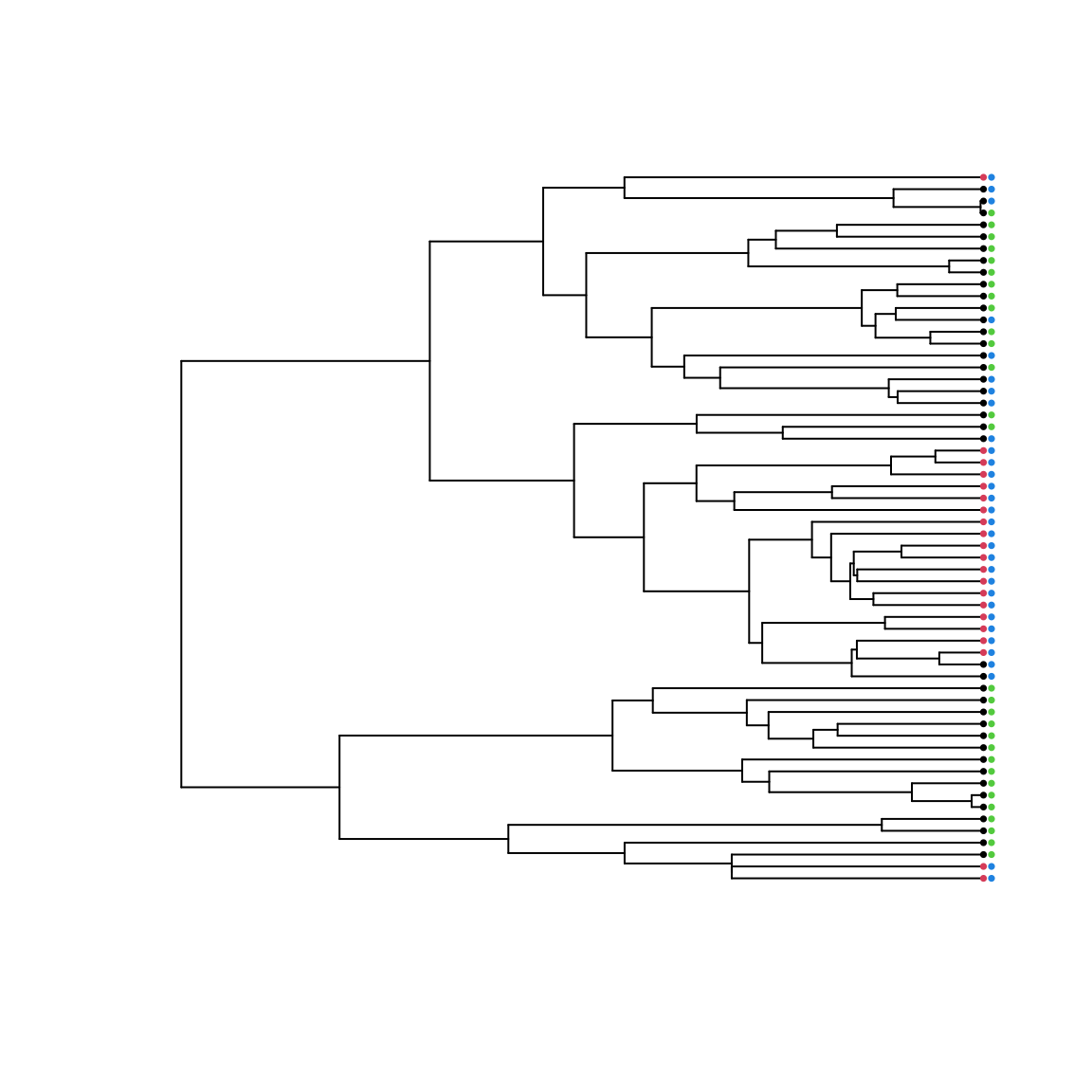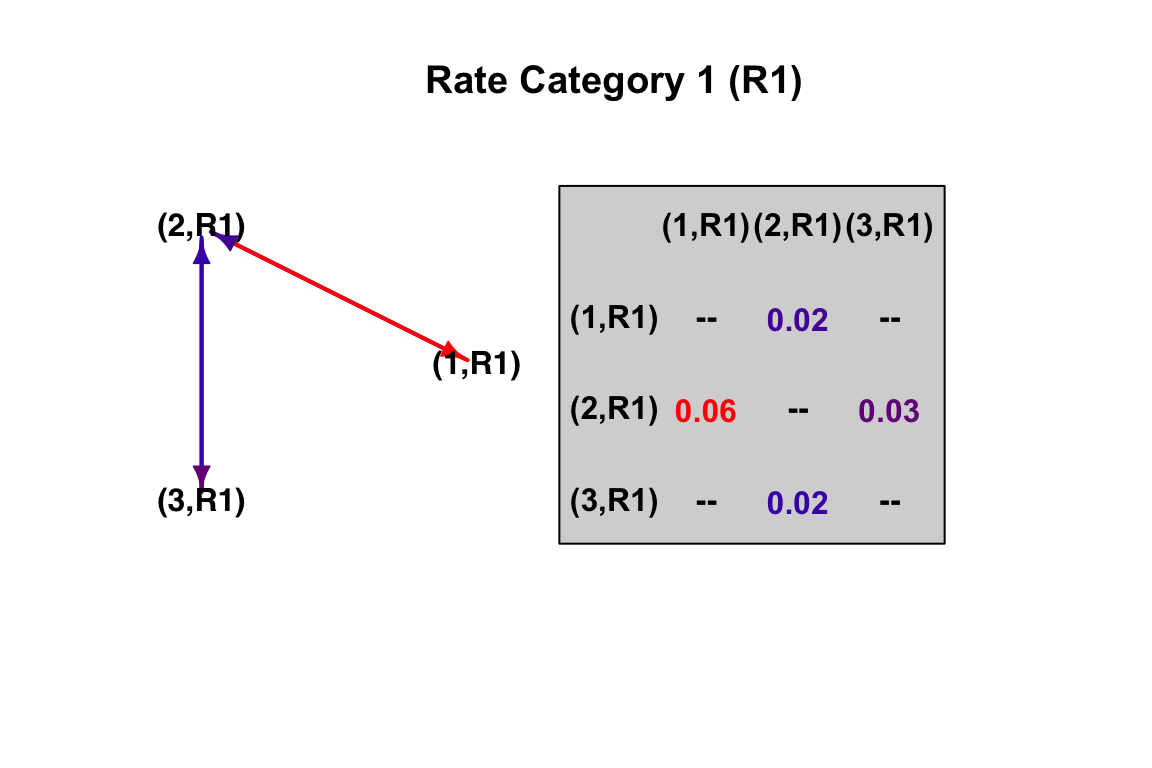corHMM 2.1: Generalized hidden Markov models

The vignette is comprised of three sections, where we demonstrate all new extensions as well as other new and useful features:

• Background information

• Section 1 Default use of corHMM

• 1.1: No hidden rate categores
• 1.2: Any number of hidden rate categories
• Section 2 How to make and interpret custom models

• 2.1: Creating and using custom rate matrices
• 2.1.1: One rate category
• 2.1.2: Any number of rate categories
• 2.2: Some examples of “biologically informed” models
• 2.2.1: Ordered habitat change
• 2.2.2: Precursor model
• 2.2.3: Ontological relationship of multiple characters
• Section 3 Estimating models when node states are fixed

• 3.1: Fixing a single node
• 3.2: Estimating rates under a parsimony reconstruction
• 3.3: Fixing nodes when the model contains hidden states

#Background information

The original version of corHMM contained a number of distinct functions for conducting analyses of discrete morphological characters. This included the corHMM() function for fitting a hidden rates model, which uses “hidden” states as a means of allowing transition rates in a binary character to vary across a tree. In reality, the hidden rates model falls within a general class of models, hidden Markov models (HMM), that may also be applied to multistate characters. So, whether the focal trait is binary or contains multiple states, or whether the observed states represents a set of binary and multistate characters, hidden states can be applied as a means of allowing heterogeneity in the transition model. Choosing a model specific to your question is of utmost importance in any comparative method, and in this new version of corHMM we provide users with the tools to create their own hidden Markov models.

Before delving into this further it may be worth showing a little of what is underneath the hood. To begin, consider a single binary character with states 0 and 1. If the question was to understand the asymmetry in the transition between these two states, the model, Q, would be a simple 2x2 matrix,

$Q= \begin{bmatrix} - & q_{0 \rightarrow 1} \\ q_{1 \rightarrow 0} & - \\ \end{bmatrix}$ This transition rate matrix is read as describing the transition rate from ROW to COLUMN. Thus, there are only two states, 0 and 1, and two transitions going from 0 $$\rightarrow$$ 1, and from 1 $$\rightarrow$$ 0. However, if we introduce a second binary character, the number of possible states you could observe is expanded to account for all the combination of states between two characters – that is, you could observe 00, 01, 10, or 11. To accommodate this, we need to expand our model such that it becomes a 4x4 matrix,

$Q = \begin{bmatrix} - & q_{00 \rightarrow 01} & q_{00 \rightarrow 10} & q_{00 \rightarrow 11}\\ q_{01 \rightarrow 00} & - & q_{01 \rightarrow 10} & q_{01 \rightarrow 11}\\ q_{10 \rightarrow 00} & q_{10 \rightarrow 01} & - & q_{10 \rightarrow 11}\\ q_{11 \rightarrow 00} & q_{11 \rightarrow 01} & q_{11 \rightarrow 10} & -\\ \end{bmatrix}$

This model is considerably more complex, as the number of transitions in this rate matrix now goes from 2 to 12. However, with these models we often make a simplifying assumption that we do not allow for transitions in two states to occur at the same time. In other words, if a lineage is in state 00 it must first transition to either state 01 or 10, before transitioning to state 11. Therefore, we can simplify the matrix by removing these “dual” transitions from the model completely,

$Q = \begin{bmatrix} - & q_{00 \rightarrow 01} & q_{00 \rightarrow 10} & -\\ q_{01 \rightarrow 00} & - & - & q_{01 \rightarrow 11}\\ q_{10 \rightarrow 00} & - & - & q_{10 \rightarrow 11}\\ - & q_{11 \rightarrow 01} & q_{11 \rightarrow 10} & -\\ \end{bmatrix}$

What we just described is the popular model of Pagel (1994), which tests for correlated evolution between two binary characters. But, one thing that is not obvious: the states in the model need not be represented as combinations of binary characters. For example, the focal character may be two characters, like say, flowers that are red with and without petals, and blue flowers with and without petals. One could just code it as a single multistate character, where 1=red petals, 2=red with no petals (i.e., sepals are red), 3=blue petals, and 4=blue with no petals (i.e., sepals are blue). The model would then be,

$Q = \begin{bmatrix} - & q_{1 \rightarrow 2} & q_{1 \rightarrow 3} & q_{1 \rightarrow 4}\\ q_{2 \rightarrow 1} & - & q_{2 \rightarrow 3} & q_{2 \rightarrow 4}\\ q_{3 \rightarrow 1} & q_{3 \rightarrow 2} & - & q_{3 \rightarrow 4}\\ q_{4 \rightarrow 1} & q_{4 \rightarrow 2} & q_{4 \rightarrow 3} & -\\ \end{bmatrix}$

Notice it is the same as before, but the states are transformed from binary combinations to a multistate character. As before, we may assume that transitions in two states cannot occur at the same time and remove the “dual” transitions.

$Q = \begin{bmatrix} - & q_{1 \rightarrow 2} & q_{1 \rightarrow 3} & -\\ q_{2 \rightarrow 1} & - & - & q_{2 \rightarrow 4}\\ q_{3 \rightarrow 1} & - & - & q_{3 \rightarrow 4}\\ - & q_{4 \rightarrow 2} & q_{4 \rightarrow 3} & -\\ \end{bmatrix}$

Again, exactly the same.

The updated version of corHMM() now lets users transform a set of characters into a single multistate character. This means that two characters need not have the same number of character states – that is, one trait could have four states, and the other could be binary. We also allow any model to be expanded to accomodate an arbitrary number of hidden states. Thus, corHMM() is completely flexible and naturally contains rayDISC() and corDISC() capabilities - standalone functions in previous versions of corHMM that may have been mistaken as different “methods.” As this vignette will show, they are indeed nested within a broader class of HMMs.

# Section 1: Default use of corHMM

## 1.1: No hidden rate categories

To start, we’ll use the primate dataset from Pagel and Meade (2006) that comes with corHMM:

set.seed(1985)
require(ape)
require(expm)
require(corHMM)
data(primates)
phy <- primates[]
phy <- multi2di(phy)
data <- primates[]
plot(phy, show.tip.label = FALSE)
data.sort <- data.frame(data[, 2], data[, 3], row.names = data[,1])
data.sort <- data.sort[phy$tip.label, ] tiplabels(pch = 16, col = data.sort[,1]+1, cex = 0.5) tiplabels(pch = 16, col = data.sort[,2]+3, cex = 0.5, offset = 0.5)We have two characters each with two possible states: trait 1 is the absence (black) or presence (red) of estrus advertisement in females, and trait 2 is single male (green) or multimale (blue) mating system in primates. The default use of corHMM() only requires that you declare your phylogeny, your dataset, and the number of rate categories (more detail about this later). We have updated corHMM() to handle different types of input data. Now to use corHMM(), the first column must be species names (as in the previous version), but there can be any number of data columns. If your dataset does have 2 or more columns of trait information, each column is taken to describe a separate character. Note that when the corHMM() call is used, the function automatically determines all the unique character combinations observed in the data set. In our primate example only 3 of the 4 possible combinations are observed, and so the model is constructed accordingly. Also, dual transitions are automatically disallowed. In other words, we expect that a species cannot go directly from estrus advertisement being absent in a single male mating system to having estrus advertisement in a multimale mating system. They must first evolve either estrus advertisement or multimale mating system. Let’s give this a try: ## MK_3state <- corHMM(phy = phy, data = data, rate.cat = 1) load("corHMMResults.Rsave") MK_3state ## ## Fit ## -lnL AIC AICc Rate.cat ntax ## -41.90867 91.81735 92.54462 1 60 ## ## Legend ## 1 2 3 ## "0_0" "0_1" "1_1" ## ## Rates ## (1,R1) (2,R1) (3,R1) ## (1,R1) NA 0.01899834 NA ## (2,R1) 0.05663462 NA 0.02627072 ## (3,R1) NA 0.01610455 NA ## ## Arrived at a reliable solution When you run your corHMM object you are greeted with a summary of the model. Your model fit is described by the log likelihood (lnL), Akaike information criterion (AIC), and sample size corrected Akaike information criterion (AICc). You are also given the number of rate categories (Rate.cat) and number of taxa (ntax). The Rates section of the output describes transition rates between states and are organized as a matrix. Again, the transition rate matrix is read as the transition rate from ROW to COLUMN. For example, if you were interested in the transition rate from State 1 (i.e., absence of estrus advertisement in a single male mating system) to State 2 (i.e., absence of estrus advertisement in a multimale mating system) you would be looking at the Row 1, Column 2, entry. For a time calibrated ultrametric tree, these rates will depend on the age of your phylogeny. You may also notice that corHMM() printed a state legend to the screen. Thus, you can obtain the exact coding for each species in an augmented dataframe provided by the corHMM() results object itself. This dataframe uses the initial user data to create columns that corresponds to how each species was represented in corHMM(): head(MK_3state$data.legend)

Alternatively, a user can supply their dataset to getStateMat4Dat, which outputs a legend that is consistent with the corHMM() function. The other output is an index matrix (or rate matrix) that describes which rates are to be estimated in corHMM(). We provide an in-depth discussion about this part of the index matrix later:

getStateMat4Dat(data)
## $legend ## 1 2 3 ## "0_0" "0_1" "1_1" ## ##$rate.mat
##     (1) (2) (3)
## (1)   0   2   0
## (2)   1   0   4
## (3)   0   3   0

Finally, interpreting a Markov matrix can be difficult, especially when you’re just starting out. This problem is compounded when users begin to apply the more complex hidden Markov models (i.e. setting rate.cat > 1). To help users, we have implemented a new plotting function:

plotMKmodel(MK_3state)This function uses a corHMM object (which is the result of running corHMM()) or a custom rate matrix (discussed in a later section) to plot the model in two parts. On the left is a ball and stick diagram that depicts the state transitions. On the right is a simplified rate matrix that contains rounded values from the solution output of corHMM(). The colors of the arrows correspond to the magnitude of the rates.

The final new plotting tool we have made available to users is a stochastic character mapping function, makeSimmap (Bollback, 2006). We can use makeSimmap to create a character history for any corHMM model and then use plotSimmap (from the popular R-package, phytools) to plot the output.

phy = MK_3state$phy data = MK_3state$data
model  = MK_3state\$solution
model[is.na(model)] <- 0
diag(model) <- -rowSums(model)
# run get simmap (can be plotted using phytools)
simmap <- makeSimmap(tree=phy, data=data, model=model, rate.cat=1, nSim=1, nCores=1)
# we import phytools plotSimmap for plotting
phytools::plotSimmap(simmap[], fsize=.5)
## no colors provided. using the following legend:
##       0_0       0_1       1_1
##   "black" "#DF536B" "#61D04F"